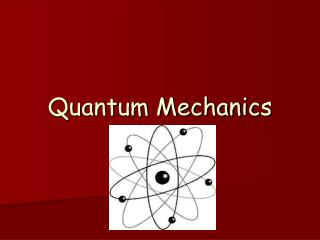DownloadDownload PresentationQuantum Mechanics

# Quantum Mechanics

Télécharger la présentation## Quantum Mechanics

- - - - - - - - - - - - - - - - - - - - - - - - - - - E N D - - - - - - - - - - - - - - - - - - - - - - - - - - -
##### Presentation Transcript

1. Quantum Mechanics

2. Background • Remember, Bohr said the energy of electrons is quantized (only have specific amount of energy) • Movement of electrons is how electromagnetic radiation, including light, is produced • Bohr’s model only works for hydrogen 

3. Background • Light can have properties of waves & particles (electrons, too) • If electrons were wavelike & restricted to certain orbits of fixed size, only certain frequencies & energies would be allowed

4. Background • Schrödinger provided a mathematical model with an equation to explain electron behavior as waves • Said location of an electron could be found with 90% accuracy

5. Background • Electrons can be studied as particles or waves, but not both at the same time • Scientists struggled to more accurately provide exact location of a moving electron • The problem was finally solved… position & momentum of a moving object (an electron) cannot be measured & known exactly (Heisenberg’s Uncertainty Principle)

6. Quantum Mechanical Model • Modern day model of the atom – The Quantum Mechanical Model • Electrons exist in orbitals – regions around a nucleus of an atom where an e- with a given energy is likely to be found • Orbitals have characteristic shapes, sizes, and energies but do not describe how the e- actually moves • Principal Energy Levels (n) - Sublevels (s, p, d, f) - Orbitals - Electrons (spin clockwise or counterclockwise)

7. Quantum Mechanical Model

8. Quantum Mechanical Model • Electrons can be found in various orbitals about 90% of the time • S sublevel has 1 orbital, P has 3 orbitals – Px, Py, Pz

9. Quantum Mechanical Model • d sublevels